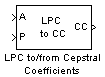# LPC to/from Cepstral Coefficients

Convert linear prediction coefficients to cepstral coefficients or cepstral coefficients to linear prediction coefficients

## Library

Estimation / Linear Prediction

`dsplp`

•## Description

The LPC to/from Cepstral Coefficients block either converts linear prediction coefficients (LPCs) to cepstral coefficients (CCs) or cepstral coefficients to linear prediction coefficients. Set the Type of conversion parameter to `LPCs to cepstral coefficients` or ```Cepstral coefficients to LPCs``` to select the domain into which you want to convert your coefficients. The LPC port corresponds to LPCs, and the CC port corresponds to the CCs. For more information, see Algorithm.

The block input can be an N-by-M matrix or an unoriented vector. Each column of the matrix is treated as a channel. When the input is an unoriented vector, the input is treated as one channel.

Consider a signal x(n) as the input to an FIR analysis filter represented by LPCs. The output of this analysis filter, e(n), is known as the prediction error signal. The power of this error signal is denoted by P, the prediction error power.

When you select `LPCs to cepstral coefficients` from the Type of conversion list, you can specify the prediction error power in two ways. From the Specify P list, choose `via input port` to input the prediction error power using input port P. The input to the port must be a vector with length equal to the number of input channels. Select `assume P equals 1` to set the prediction error power equal to 1 for all channels.

When you select `LPCs to cepstral coefficients` from the Type of conversion list, the Output size same as input size check box appears. When you select this check box, the length of the input vector of LPCs is equal to the output vector of CCs. When you do not select this check box, enter a positive scalar for the Length of output cepstral coefficients parameter.

When you select `LPCs to cepstral coefficients` from the Type of conversion list, you can use the If first input value is not 1 parameter to specify the behavior of the block when the first coefficient of the LPC vector is not 1. The following options are available:

• `Replace it with 1` —- Changes the first value of the coefficient vector to 1. The other coefficient values are unchanged.

• `Normalize` — Divides the entire vector of coefficients by the first coefficient so that the first coefficient of the LPC vector is 1.

• `Normalize and Warn` — Divides the entire vector of coefficients by the first coefficient so that the first coefficient of the LPC vector is 1. The block displays a warning message telling you that your vector of coefficients has been normalized.

• `Error` — Displays an error telling you that the first coefficient of the LPC vector is not 1.

When you select `Cepstral coefficients to LPCs` from the Type of conversion list, the Output P check box appears on the block. Select this check box when you want to output the prediction error power from output port P.

## Algorithm

The cepstral coefficients are the coefficients of the Fourier transform representation of the logarithm magnitude spectrum. Consider a sequence, x(n), having a Fourier transform X(ω). The cepstrum, cx(n), is defined by the inverse Fourier transform of Cx(ω), where Cx(ω) = logeX (ω). See the Real Cepstrum block reference page for information on computing cepstrum coefficients from time-domain signals.

### LPC to CC

When in this mode, this block uses a recursion technique to convert LPCs to CCs. The LPC vector is defined by $\left[\begin{array}{cccc}{a}_{0}& {a}_{1}& {a}_{2}& \begin{array}{cc}...& {a}_{p}\end{array}\end{array}\right]$ and the CC vector is defined by $\left[\begin{array}{ccccccc}{c}_{0}& {c}_{1}& {c}_{2}& ...& {c}_{p}& ...& {c}_{n-1}\end{array}\right]$. The recursion is defined by the following equations:

`${c}_{0}={\mathrm{log}}_{e}P$`
`${c}_{m}=-{a}_{m}+\frac{1}{m}\sum _{k=1}^{m-1}\left[-\left(m-k\right)\cdot {a}_{k}\cdot {c}_{\left(m-k\right)}\right],1\le m\le p$`
`${c}_{m}=\sum _{k=1}^{p}\left[\frac{-\left(m-k\right)}{m}\cdot {a}_{k}\cdot {c}_{\left(m-k\right)}\right],p`

### CC to LPC

When in this mode, this block uses a recursion technique to convert CCs to LPCs. The CC vector is defined by $\left[\begin{array}{cccc}\begin{array}{cccc}{c}_{0}& {c}_{1}& {c}_{2}& ...\end{array}& {c}_{p}& ...& {c}_{n}\end{array}\right]$ and the LPC vector is defined by $\left[\begin{array}{cccc}{a}_{0}& {a}_{1}& {a}_{2}& \begin{array}{cc}...& {a}_{p}\end{array}\end{array}\right]$. The recursion is defined by the following equations

`${a}_{m}=-{c}_{m}-\frac{1}{m}\sum _{k=1}^{m-1}\left[\left(m-k\right)\cdot {c}_{\left(m-k\right)}\cdot {a}_{k}\right]$`

`$P=\mathrm{exp}\left({C}_{0}\right)$`

where $m=1,2,...,p$.

## Parameters

Type of conversion

Choose `LPCs to cepstral coefficients` or `Cepstral coefficients to LPCs` to specify the domain into which you want to convert your coefficients.

Specify P

Choose `via input port` to input the values of prediction error power using input port P. Select ```assume P equals 1``` to set the prediction error power equal to 1.

Output size same as input size

When you select this check box, the length of the input vector of LPCs is equal to the output vector of CCs.

Length of output cepstral coefficients

Enter a positive scalar that is the length of each output channel of CCs.

If first input value is not 1

Select what you would like the block to do when the first coefficient of the LPC vector is not 1. You can choose ```Replace it with 1```, `Normalize`, `Normalize and Warn`, and `Error`.

Output P

Select this check box to output the prediction error power for each channel from output port P.

## References

Papamichalis, Panos E. Practical Approaches to Speech Coding. Englewood Cliffs, NJ: Prentice Hall, 1987.

## Supported Data Types

• Double-precision floating point

• Single-precision floating point